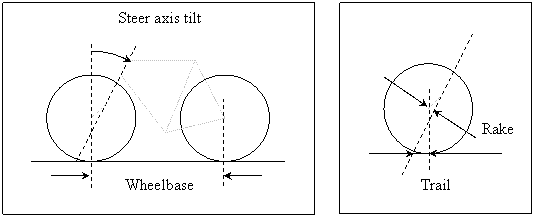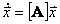JBike6 Benchmarking

Benchmarking a Fully Non-Linear Bicycle Model with JBike6

The Biorobotics and Locomotion Laboratory

Department of Theoretical and Applied Mechanics

Cornell University, Ithaca, NY

2003-2015

Abstract

The linearized equations of motion implemented in JBike6 have been exhaustively verified in paper by A. L. Schwab, J. P. Meijaard, and J. M. Papadopoulos.

“The entries of the matrices of these equations form the basis for comparison. Three different kinds of methods to obtain the results are compared: pencil-and-paper [the equations used in JBike6], the numeric multibody dynamics program SPACAR, and the symbolic software system AutoSim. Because the results of the three methods are the same within the machine round-off error, we assume that the results are correct and can be used as a bicycle dynamics benchmark.”

Thus, they serve as an excellent benchmark to verify any other bicycle equations of motion. Here, we show how to do exactly that.Contents

Introduction

# Introduction

The advent of cheap and plentiful computer power and numerical methods provides the opportunity to simulate bicycle motion from fully non-linear equations. Although systematic methods exist for generating these equations that avoid the complex assumptions and simplification required for linearized equations, the question of their accuracy remains.

Fortunately, a way exists to confirm fully non-linear equations: comparison with a known benchmark’s eigenvalues. They are intrinsic to the modeled system and are completely independent of coordinates and units chosen.

JBike6 provides these eigenvalues from its linearized equations. Here we show how to extract them from the non-linear equations, and compare the results.

# The Bicycle Model

In both cases the bicycle model consists of:

·        4 rigid bodies in 3 dimensions all with left-right mass symmetry.

·        Connected by 3 revolute or pin joints: steering axis and two wheel hubs.

·        Rider is rigidly attached to the frame (optional front and rear racks also rigidly attached).

·        No friction at any joint.

·        Wheels are knife-edged disks with left-right mass symmetry and polar mass symmetry, and they roll without slip or loss due to friction.

·        Ground is a rigid, flat, horizontal surface.

·        Only external forces are gravity, the ground reaction.

·        Always starts in an upright, straight ahead configuration with some given forward velocity.

·        Two non-holonomic constraints, one at each wheel contact point.

Key parameter definitions:

###### ·        Wheelbase: distance between wheel-ground contact points.

·        Steering axis tilt: amount steering axis is tilted from the vertical.

###### ·        Trial: distance from front wheel ground contact point to point where steering axis intersects the ground.

·        Rake: amount fork is offset, usually forward in order to reduce trail. (Redundant and not used, but included for completeness.)# Linearized Equations

Only three coordinates are necessary to specify a bicycle on a plane:

·        Lean angle

·        Steer angle

·        Forward position of rear wheel.

However, the acceleration is taken to be zero to the first order and so the forward velocity is constant. This leaves only two non-trivial degrees of freedom: lean and steer angle. Two coupled second-order ordinary differential equations represent the motion of the system.

In the actual derivation for his Masters Thesis, Scott Hand used constrained Lagrange equations and then eliminated the constraints.

The Equations of Motionwhere

·is the lean angle of the rear assembly

·is the steer angle of the front assembly relative to the rear assembly

·        Mθ and Mψ are moments (torques) applied at the rear assembly and the steering axis, respectively, and which are both taken to be zero.

# Non-linear Equations

The four rigid bodies used to model a bicycle (the same as for the linearized model) with the mass and inertia of the rear rack combined with that of the rear frame and the mass and inertia of the front basket combined with that of the front fork.The Coordinates are:

4 bodies in 3-D:

4 x 3 = 12 position coordinates

4 x 3 = 12 orientation coordinates (angles)

24 total coordinates

3 revolute joints:

3 x 3 = 9 position coincident constraints

3 x 2 = 6 axis parallel constraints

2 ground contact points:

2 x 1 = 2 position constraints (vertical contact)

2 x 2 = 4 non-holonomic velocity constraints (wheels roll without slip)

17 coordinate constraints and 4 velocity constraintswhereis the 3-D position vector of body i in space-fixed coordinates: (xi, yi, zi)is the orientation vector of body i in space-fixed Euler angles: ()is the 3-D velocity vector of body i in space-fixed coordinatesis the angular velocity vector of body i in body-fixed coordinateswhere D is the 'Jacobian' of the velocity constraints and gi are the 'convective' terms, and since I3 is used to represent the 3x3 identity, the body-fixed inertia matrix for body i is written J'i.

# Numerical Implementation

Written in MATLAB version 6.5 from The MathWorks

With numerical integration via RK4, although integration is not used in the calculation of eigenvalues.

And adjusting positions and velocities back to 'constraint surface' after each numerical integration step:

·        Adjust positions by iterating with Coordinate Projection Method

·        Minimize error and smooth results by forcing at least one iteration. Without this technique, error in the position constraints builds over time and then requires a 'jarring' correction that shows up in the running energy calculations. It appears that this technique better keeps the error in position constraints under control so that multiple steps are rarely, if ever, required.

·        Adjust velocities with Pseudo inverse in one step.

# Parameters

Use in both implementations: 'Schwinn Crown'. Units are meters, kilograms, and radians.

wheelbase = 1.01600000;

trail = 0.09096800;

% rear wheel

Drearwheel = 0.68580000;

mrearwheel = 1.81818200;

Irearwheel = [0.08551300 0.08551300 0.17102600];

alphaIrearwheel = 0.00000000;

% front wheel

Dfrontwheel = 0.68580000;

mfrontwheel = 1.81818200;

Ifrontwheel = [0.08551300 0.08551300 0.17102600];

alphaIfrontwheel = 0.00000000;

% rider

mrider = 80.00000000;

xcmrider = 0.30000000;

ycmrider = 1.20000000;

Irider = [10.53112900 2.46887100 12.00000000];

alphaIrider = -0.25957299;

% frame

mframe = 2.50000000;

xcmframe = 0.30000000;

ycmframe = 0.50000000;

Iframe = [0.05857900 0.34142100 0.40000000];

alphaIframe = 0.39269899;

% front fork

mfork = 1.50000000;

ucmfork = 0.00000000;

vcmfork = 0.68580000;

Ifork = [0.05879000 0.00058800 0.05879000];

alphaIfork = 0.33200000;

% rear rack

mrack = 2.00000000;

xcmrack = -0.10000000;

ycmrack = 0.80000000;

Irack = [0.02000000 0.02000000 0.02000000];

alphaIrack = 0.00000000;

# Eigenvalues from linearized equationswhere M is the mass matrix, C is the damping matrix, K is the stiffness matrix, and D is the differential operator. Note that for the upright bike Cθθ = 0, and so it did not appear above in the equations of motion.

Combine the M, C, and K matrices into 2 4x4 matrices, A and B,andand calculate the 4 generalized eigenvalues of them via:

Ax = λBx

Where the values of λ that satisfy the equation are the generalized eigenvalues and the corresponding values of x are the generalized right eigenvectors.

In MATLAB:

B = [M zeros(2); zeros(2) eye(2)];

A = [-C -K; eye(2) zeros(2)];

[V,D] = eig(A, B);

Screen shot of JBike6 program showing the bicycle parameters, a stylized drawing of the bicycle, and the calculated eigenvalues for a range of forward speeds.# Eigenvalues from non-linear equationsand assume a solution of the form.

Then the eigenvalues, λ, of the matrix [A] describe stability: stable for λ < 0, unstable otherwise.

To construct this matrix [A] for a nonlinear system being solved numerically, recognize that the function to compute the accelerations (called by the ode solver, RK4 in this case) has the form.

Thus the matrix we seek is merely.

However,is not some function that we can simply differentiate symbolically.

Instead, to calculate partial derivatives numerically, use the definition of the derivative:(for forward differencing, with error O(ε))(or even better: centered differencing, with error O(ε2))

If access to calculated accelerations is available (which in this case is the output of the function called by the ode solver) thenIn order to select the best value for epsilon, we simply iterate until convergence.

The value of epsilon for each state variable and forward speed is chosen in a loop in which it is decimated until the resulting candidate values for a column in the matrix converge. The best value turns out to be 1e-11.

The eigenvalues of this matrix [A] can be compared to the eigenvalues from the linearized equations. In this particular case, because the non-linear equations use 48 state variables instead of the 4 used in the linearized equations to represent the 2 non-trivial degrees of freedom in the system, 48 values are generated. 4 of them correspond to the 2 non-trivial degrees of freedom in the system, and can be compared to the 4 generated from the linearized equations.

In MATLAB:

speed = 1;                  % index this as number 1

while v0 <= v0_max          % while there are more forward speeds to use

for sv = 1: state_variables  % for each state variable

e = zeros(state_variables, 1);  % initialize an epsilon vector

e(sv, 1) = epsilon;             % set one value to non-zero

for direction = -1:2:1          % for each dir for center diff

z(:, n) = z_0 + (epsilon .* direction);  % add epsilon to st v

% Apply constraints to the four bodies

%   iterate to adjust current positions subject to constraints

%   adjust current velocities subject to constraints

% Calculate accelerations (Left Hand Side)/(Right Hand Side)

[z(:, n+1), lambdas(:, n+1)] = ode(z(:, n), M, Mo_b, f, r_b);

if direction == -1

f_z_0_m_e = z(:, n+1);  % f(z_0 – epsilon)

else

f_z_0_p_e = z(:, n+1);  % f(z_0 + epsilon)

A(:, sv) = (f_z_0_p_e - f_z_0_m_e) / (2 * epsilon);  % add

end

end

end

[V,D] = eig(A);           % calculate eigenvalues

eigs(:, speed) = diag(D); % store them away

v0s(speed) = v0;          % store forward speed

v0 = v0 + v0_step;        % increment forward speed

speed = speed + 1;        % increment index

end

# Results

The plots below show the excellent match of both real and imaginary parts of eigenvalues from the two methods.The plots below show the difference between just the real parts of the values from the two methods.at 10-6at 10-7at 10-8at 10-5 and only the part of the forward speed range near the capsize speed.

Maximum difference between the two methods occurs near the capsize speed (7.9807 m/s), where an eigenvalue crosses the real axis from negative to positive. The difference peaks at just under 1.5e-05.

Another, smaller increase in error occurs just before (forward speeds < 1 m/s) a double root: a bifurcation where two eigenvalues with different real parts and zero imaginary parts approach the same value and then (for higher forward speeds) have the same real parts and complimentary imaginary parts. There the difference peaks at just over 1e-07.

In all other cases, the difference between eigenvalues from the two different methods remains less than 6e-08.

# Conclusions

The non-linear equations of motion are confirmed.

# Future Work

Investigate and eliminate spike in difference between eigenvalues from linearized and non-linear equations when velocity is near 8 m/s.

Investigate generating more accurate eigenvalues from non-linear model with more accurate derivatives.

# Acknowledgements

Andy Ruina, Professor of Theoretical and Applied Mechanics at Cornell University, chief of the The Biorobotics and Locomotion Lab, and my advisor.

Arend Schwab, Assistant Professor in Applied Mechanics at of Delft University of Technology and creator of TAM 674: Applied Multibody Dynamics, the class in which we derived the non-linear bicycle model and implemented it in MATLAB. Also co-author of JBike6.

Jim Papadopoulos, author of JBike5 and co-author of JBike6

The rest of the Theoretical & Applied Mechanics Biorobotics and Locomotion Lab at Cornell University: Mario Gomes, Dave Cabrera, Manoj Srinivasan, and Sam Walcott.

Fellow T & AM graduate student Geoff Recktenwald.

# Simulation

Here are some video clips of the animated simulation confirmed by JBike6.

# Footnotes

 ] Benchmark Results on the Linearized Equations of Motion of an Uncontrolled Bicycle, 2004, A. L. Schwab, J. P. Meijaard, and J. M. Papadopoulos, page 1.

 Hand Masters Thesis, Cornell, 1988, page 23.

 page 35.

 Schwab class notes, 2003

 Papadopoulos and Schwab JBike6, 2003

 Ruina, 2004